How to determine divisibility in Excel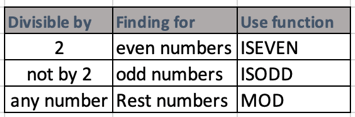In this article, we will learn How to determine divisibility in Excel.

Scenario:

Divisible is a mathematical operation performed between two numbers. It returns two values quotient and remainder. The given two numbers are divisor and Dividend. Like when 100 is divided by 15. It returns 6 as quotient and 10 as remainder. 15 times 6 is 90 and 90 + 10 equals 100. Let's understand it better with the operation performed below.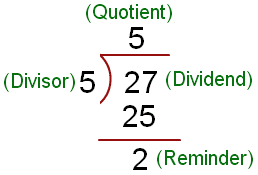So it becomes

Dividend or number = divisor * quotient + remainder

For a division to happen we need Dividend and divisor, all rest are calculated. We can get Quotient value just by using the divide ( / ) operator. But for the remainder value we can use the MOD function in Excel. For example we get quotient 5 by using =27/5 in Excel cells. And calculator the remainder by = 27 - (27/5) * 5 = 2 (remainder). Or you can use =MOD(27,5) returns 2

Here we are just focused on the remainder value. So Let's get around it using the Excel functions here.

Divisible by 2 in excel

Divisibility by 2 means the number is an even number. In mathematical terms when a number is divided by 2 and it returns 0. So the number is divisible by 2 and is an even number. In Excel use the ISEVEN function to find the even numbers in the list.

Formula :

 =ISEVEN(number)

The above function returns TRUE or FALSE

Not Divisible by 2 in excel

Not Divisible by 2 means the number is an Odd number. In mathematical terms when a number is divided by 2 and returns 1 . So the number is not divisible by 2 and is an odd number. In Excel use the ISODD function to find the even numbers in the list.

Formula :

 =ISODD(number)

The above function returns TRUE or FALSE

Divisible by any other number

You can use this method to check divisibility of any number by any number. MOD function in Excel returns the integer remainder value. So the below formula checks if the MOD function returns 0 (divisible), the formula returns True or if the MOD function returns any other value than 0 then the formula returns FALSE.

Formula :

 =MOD(Dividend,divisor)=0

MOD function returns remainder value but the formula checks if its 0 or not.

Example :

Divisible by 2

All of these might be confusing to understand. Let's understand how to use the function using an example. Here we have some numbers to divide. First we check if the number is divisible by 2.

Use the formula:

 =ISEVEN(B1)

Copy and paste the formula to all rest values to chcek divisibility by 2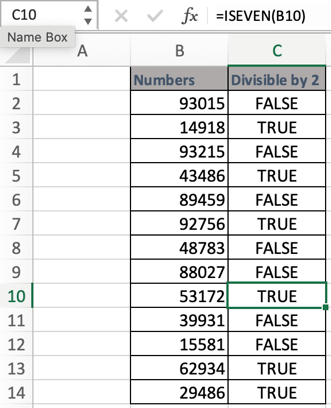All the values where TRUE is returned are even numbers and divisible by 2

Not divisible by 2

To check a number is divisible by 2 or not. As every alternate number is divisible by 2 and rest are not. So in the above example all the FALSE values are odd numbers.

Now we check if the number is divisible by 2 or not.

Use the formula:

 =ISODD(B1)

Copy and paste the formula to all rest values to check divisibility by 2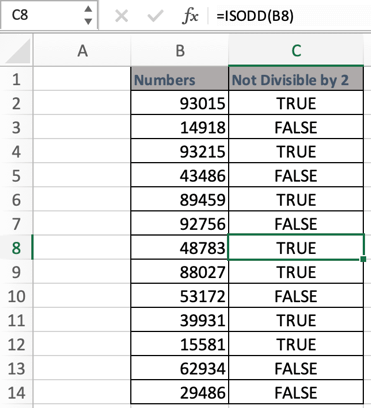All the values where TRUE is returned are odd numbers and not divisible by 2 whereas rest are even numbers.

Divisible By 15

To check divisibility of any number by 15 (random number). Just use the below formula and rest excel.

Use the formula:

 =MOD(B1,15)=0

Copy and paste the formula to all rest values to check divisibility by 2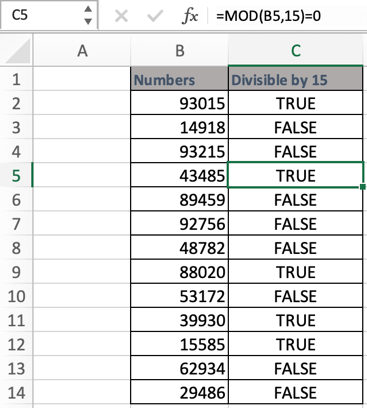All the values where TRUE is returned are divisible by 15 whereas rest are not.

Note: You can input numbers using cell reference or directly within function itself. Both are considered the same.

Hope this article about How to determine divisibility in Excel is explanatory. Find more articles on calculating values and related Excel formulas here. If you liked our blogs, share it with your friends on Facebook. And also you can follow us on Twitter and Facebook. We would love to hear from you, do let us know how we can improve, complement or innovate our work and make it better for you. Write to us at info@exceltip.com.

Related Articles :

How to use the ISEVEN function in Excel : returns the TRUE logic value if the number is EVEN using the ISEVEN function in Excel. Learn more about the ISEVEN function here

How to use the ISODD function in Excel : returns the TRUE logic value if the number is ODD using the ISODD function in Excel. Learn more about the ISODD function here

How to use the MOD function in Excel : returns the remainder value in integer. Remainder is the positive number which remains when two numbers divide. Learn more about the MOD function here.

Ignore zero in the Average of numbers : calculate the average of numbers in the array ignoring zeros using AVERAGEIF function in Excel.

Calculate Weighted Average : find the average of values having different weight using SUMPRODUCT function in Excel.

Find AVERAGE of top 3 scores in a list: Find the average of numbers with criteria as highest 3 numbers from the list in Excel

How to calculate Standard Deviation in Excel : To calculate the standard deviation we have different functions in Excel. The standard deviation is the square root of the variance value but It tells more about the dataset than variance.

How to calculate variance in Excel : Calculate the variance for the sample dataset in excel using the VAR function in Excel.

Popular Articles :